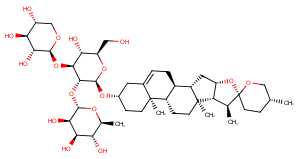# Ophiopogonin D'

Catalog No. TN1071   CAS 65604-80-0

Ophiopogonin D' can activate SIRT1 in a dose-dependent manner. Ophiopogonin D' also noncompetitively inhibits UGT1A6 and UGT1A10.

All products from TargetMol are for Research Use Only. Not for Human or Veterinary or Therapeutic Use.Ophiopogonin D', CAS 65604-80-0
Product consultation
Get quote
Purity: 98%
Biological Description
Chemical Properties
Storage & Solubility Information
 Description Ophiopogonin D' can activate SIRT1 in a dose-dependent manner. Ophiopogonin D' also noncompetitively inhibits UGT1A6 and UGT1A10. In vitro In vitro incubation system to model UGT reaction was used. Recombinant UGT isoforms-catalyzed 4-methylumbelliferone (4-MU) glucuronidation and UGT1A4-catalyzed trifluoperazine (TFP) glucuronidation reactions were employed to phenotype the inhibition profile of maidong's components towards the activity of UGT isoforms. Different inhibition potential of maidong's components towards various UGT isoforms was observed. Based on the inhibition kinetic investigation results, ophiopogonin D (OD) noncompetitively inhibited UGT1A6 and competitively inhibited UGT1A8, Ophiopogonin D' (OD') noncompetitively inhibited UGT1A6 and UGT1A10, and ruscorectal (RU) exhibited competitive inhibition towards UGT1A4. The inhibition kinetic parameters were calculated to be 20.6, 40.1, 5.3, 9.0, and 0.02 μM, respectively.
 Molecular Weight 855.02 Formula C44H70O16 CAS No. 65604-80-0

#### Storage

Powder: -20°C for 3 years

In solvent: -80°C for 2 years

#### Solubility Information

DMSO: 8.55 mg/mL (10 mM)

( < 1 mg/ml refers to the product slightly soluble or insoluble )

##Dose Conversion

You can also refer to dose conversion for different animals. More

##In vivo Formulation Calculator (Clear solution)

Step One: Enter information below
Dosage
mg/kg
Average weight of animals
g
Dosing volume per animal
ul
Number of animals
Step Two: Enter the in vivo formulation
% DMSO
%
% Tween 80
% ddH2O

##Calculator

Molarity Calculator
Dilution Calculator
Reconstitution Calculation
Molecular Weight Calculator
=
X
X

### Molarity Calculator allows you to calculate the

• Mass of a compound required to prepare a solution of known volume and concentration
• Volume of solution required to dissolve a compound of known mass to a desired concentration
• Concentration of a solution resulting from a known mass of compound in a specific volume
See Example

An example of a molarity calculation using the molarity calculator
What is the mass of compound required to make a 10 mM stock solution in 10 ml of water given that the molecular weight of the compound is 197.13 g/mol?
Enter 197.13 into the Molecular Weight (MW) box
Enter 10 into the Concentration box and select the correct unit (millimolar)
Enter 10 into the Volume box and select the correct unit (milliliter)
Press calculate
The answer of 19.713 mg appears in the Mass box

X
=
X

### Calculator the dilution required to prepare a stock solution

Calculate the dilution required to prepare a stock solution
The dilution calculator is a useful tool which allows you to calculate how to dilute a stock solution of known concentration. Enter C1, C2 & V2 to calculate V1.

See Example

An example of a dilution calculation using the Tocris dilution calculator
What volume of a given 10 mM stock solution is required to make 20ml of a 50 μM solution?
Using the equation C1V1 = C2V2, where C1=10 mM, C2=50 μM, V2=20 ml and V1 is the unknown:
Enter 10 into the Concentration (start) box and select the correct unit (millimolar)
Enter 50 into the Concentration (final) box and select the correct unit (micromolar)
Enter 20 into the Volume (final) box and select the correct unit (milliliter)
Press calculate
The answer of 100 microliter (0.1 ml) appears in the Volume (start) box

=
/

### Calculate the volume of solvent required to reconstitute your vial.

The reconstitution calculator allows you to quickly calculate the volume of a reagent to reconstitute your vial.
Simply enter the mass of reagent and the target concentration and the calculator will determine the rest.

g/mol

### Enter the chemical formula of a compound to calculate its molar mass and elemental composition

Tip: Chemical formula is case sensitive: C10H16N2O2 c10h16n2o2

Instructions to calculate molar mass (molecular weight) of a chemical compound:
To calculate molar mass of a chemical compound, please enter its chemical formula and click 'Calculate'.
Definitions of molecular mass, molecular weight, molar mass and molar weight:
Molecular mass (molecular weight) is the mass of one molecule of a substance and is expressed n the unified atomic mass units (u). (1 u is equal to 1/12 the mass of one atom of carbon-12)
Molar mass (molar weight) is the mass of one mole of a substance and is expressed in g/mol.

bottom

## Tech Support

Please see Inhibitor Handling Instructions for more frequently ask questions. Topics include: how to prepare stock solutions, how to store products, and cautions on cell-based assays & animal experiments, etc.